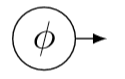# 6.3: The Frequency-Wavenumber Covector

$$\newcommand{\vecs}{\overset { \rightharpoonup} {\mathbf{#1}} }$$ $$\newcommand{\vecd}{\overset{-\!-\!\rightharpoonup}{\vphantom{a}\smash {#1}}}$$$$\newcommand{\id}{\mathrm{id}}$$ $$\newcommand{\Span}{\mathrm{span}}$$ $$\newcommand{\kernel}{\mathrm{null}\,}$$ $$\newcommand{\range}{\mathrm{range}\,}$$ $$\newcommand{\RealPart}{\mathrm{Re}}$$ $$\newcommand{\ImaginaryPart}{\mathrm{Im}}$$ $$\newcommand{\Argument}{\mathrm{Arg}}$$ $$\newcommand{\norm}{\| #1 \|}$$ $$\newcommand{\inner}{\langle #1, #2 \rangle}$$ $$\newcommand{\Span}{\mathrm{span}}$$ $$\newcommand{\id}{\mathrm{id}}$$ $$\newcommand{\Span}{\mathrm{span}}$$ $$\newcommand{\kernel}{\mathrm{null}\,}$$ $$\newcommand{\range}{\mathrm{range}\,}$$ $$\newcommand{\RealPart}{\mathrm{Re}}$$ $$\newcommand{\ImaginaryPart}{\mathrm{Im}}$$ $$\newcommand{\Argument}{\mathrm{Arg}}$$ $$\newcommand{\norm}{\| #1 \|}$$ $$\newcommand{\inner}{\langle #1, #2 \rangle}$$ $$\newcommand{\Span}{\mathrm{span}}$$$$\newcommand{\AA}{\unicode[.8,0]{x212B}}$$

Learning Objectives

• Study $$\omega \to$$ the frequency-wavelength covector

Generalizing from $$0 + 1$$ dimensions to $$3 + 1$$, we could have an observer moving inertially along velocity vector $$\to o$$, while counting the phase $$φ$$ (in radians) of a plane wave (perhaps a water wave or an electromagnetic wave) that is washing over her. Since $$φ$$ is just a count, it’s clearly a scalar. That means that we have some function that takes as its input a vector $$\to o$$ and gives as an output the scalar $$φ$$. This function has all the right characteristics to be described as a measurement $$ω\to o$$ of $$\to o$$ with some covector $$ω\to$$, and in a constructive style of mathematics this is a good way of defining a covector: it’s a linear function from the space of vectors to the real numbers. We call $$ω\to$$ the frequency-wavelength covector, or just the frequency covector for short. If $$\to o$$ represents one second as measured on the clock of this observer, then $$ω\to o$$ is the frequency $$ω$$ measured by this observer in units of radians per second. If the same observer considers $$s$$ to be a vector of simultaneity with a length of one meter, then $$ω\to s$$ is the observer’s measurement of the wavenumber $$k$$, defined as $$2π$$ divided by the wavelength.

## VisualizationFigure $$\PageIndex{1}$$: 1. A displacement vector. 2. A covector. 3. Measurement is reduced to counting. The observer, represented by the displacement vector, counts 24 wavefronts.

In more than one dimension, there are natural ways of visualizing the different vector spaces inhabited by vectors and covectors. A vector is an arrow. A covector can be visualized as a set of parallel, evenly spaced lines on a topographic map, $$\PageIndex{1}$$ (2), with an arrowhead to show which way is “uphill.” The act of measurement consists of counting how many of these lines are crossed by a certain vector, figure $$\PageIndex{1}$$ (3).

Example $$\PageIndex{1}$$: Parallelism between vectors and covectors

It seems visually obvious in figure $$\PageIndex{1}$$ (3), that the vector and covector are almost, but not exactly, parallel, since the arrowheads point in almost the same direction. Ordinarily, parallelism of nonzero vectors $$u$$ and $$v$$ would be expressed by the existence of a real number $$α$$ such that $$u = αv$$. But vectors and covectors are different kinds of beasts, belonging to different vector spaces. Scaling up a zebra will never produce a giraffe. If there is no metric, then this is simply a fact of life: there is no natural way to define parallelism between a vector $$v$$ and a covector $$ω$$.

But if we have a metric, then we can define a magnitude for the vectorc in figure $$\PageIndex{1}$$ (3), and keep that magnitude constant while rotating $$v$$. If the metric is Euclidean, then this corresponds to rigidly rotating the arrow on the page, and $$ω\to v$$ is maximized for a certain orientation, which we define as the condition for parallelism. If the metric is noneuclidean, then things get a bit more complicated, but the same ideas apply if the vectors are either both spacelike or both timelike. For example, if both are timelike, then $$ω\to v$$ is minimized by parallelism, because the Cauchy-Schwarz inequality is reversed (see section 1.5)

Given a scalar field $$φ$$, its gradient $$∇φ$$ at any given point is a covector. The frequency covector is the gradient of the phase. In birdtracks notation, we indicate this by writing it with an outwardpointing arrow, $$(∇φ)$$. Because gradients occur so frequently, birdtracks notation has a special shorthand for them, which is simply a circle:This notation can also be extended to the case where the thing being differentiated is not a scalar, but then some complications are encountered when the coordinates are not Minkowski; see Section 9.4.

Example $$\PageIndex{2}$$: Cosmological observers

Time is relative, so what do people mean when they say that the universe is $$13.8$$ billion years old? If a hypothetical observer had been around since shortly after the big bang, the time elapsed on that observer’s clock would depend on the observer’s world line. Two such observers, who had different world-lines,could have differing clock readings.

Modern cosmologists aren’t naive about time dilation. They have in mind a cosmologically preferred world-line for their observer. One way of constructing this world-line is as follows. Over time, the temperature $$T$$ of the universe has decreased. (We define this temperature locally, but we average over large enough regions so that local variations don’t matter.) The negative gradient of this temperature, $$-∇T$$, is a covector that points in a preferred direction in spacetime, and a preferred world-line for an observer is one whose velocity vector $$v$$ is always parallel to $$-∇T$$, in the sense defined in example $$\PageIndex{1}$$ above.

This page titled 6.3: The Frequency-Wavenumber Covector is shared under a CC BY-SA 4.0 license and was authored, remixed, and/or curated by Benjamin Crowell via source content that was edited to the style and standards of the LibreTexts platform; a detailed edit history is available upon request.